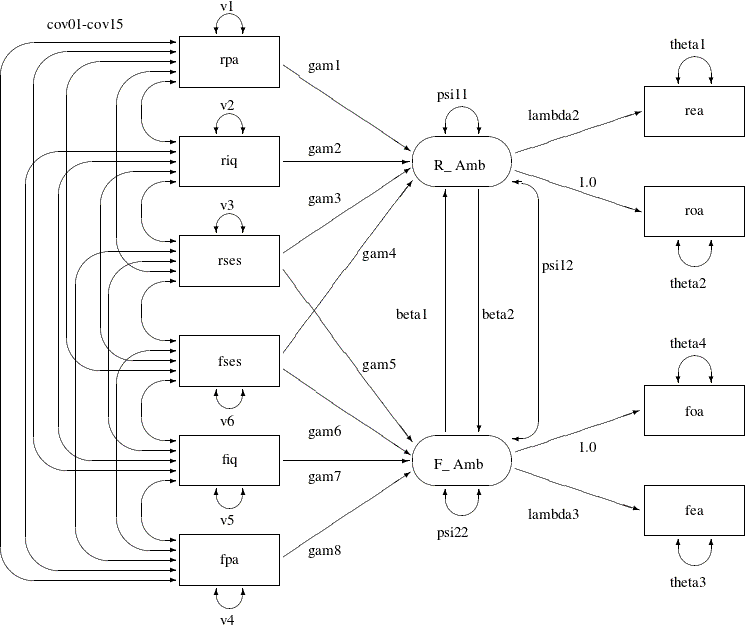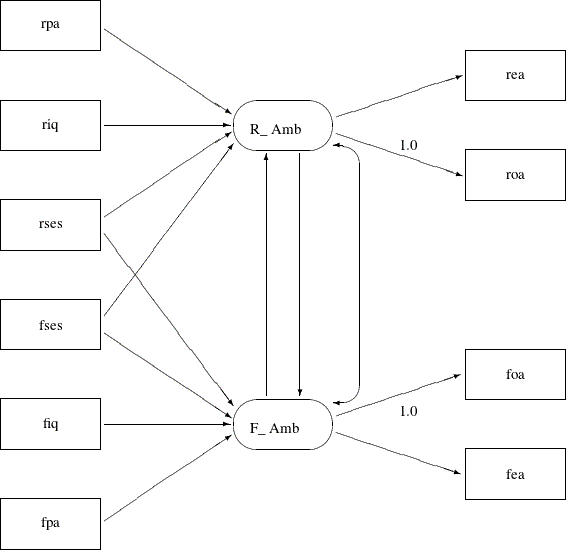### Career Aspiration: Analysis 1

The model analyzed by Jöreskog and Sörbom (1988) is displayed in the path diagram in Figure 17.36.

Figure 17.36: Path Diagram: Career Aspiration – Jöreskog and Sörbom (1988)Two latent variables, R_Amb and F_Amb, represent the respondent’s level of ambition and his best friend’s level of ambition, respectively. The model states that the respondent’s ambition (R_Amb) is determined by his intelligence (riq) and socioeconomic status (rses), his perception of his parents’ aspiration for him (rpa), and his friend’s socioeconomic status (fses) and ambition (F_Amb). It is assumed that his friend’s intelligence (fiq) and parental aspiration (fpa) affect the respondent’s ambition only indirectly through the friend’s ambition (F_Amb). Ambition is indicated by the manifest variables of occupational (roa) and educational aspiration (rea), which are assumed to have uncorrelated residuals. The path coefficient from ambition to occupational aspiration is set to 1.0 to determine the scale of the ambition latent variable.

The path diagram shown in Figure 17.36 appears to be very complicated. Sometimes when researchers draw their path diagram with a lot of variables, they omit the covariances among exogenous variables for overall clarity. For example, the double-headed paths that represent cov01cov15 in Figure 17.36 can be omitted. In addition, unconstrained variance and error variance parameters in the path diagram could be omitted without losing the pertinent information in the path diagram. For example, variance parameters v1v6 and error variance parameters theta1theta4 in Figure 17.36 detract from the main focus on the functional relationships of the model.

These omissions in the path diagram are in fact inconsequential when you transcribe them into the PATH model in PROC CALIS. The reason is that PROC CALIS employs several useful default parameterization rules that make the model specification process much easier and more intuitive. Here are the sets of default covariance structure parameters in the PATH modeling language:

• variances for all exogenous (observed or latent) variables

• error variances of all endogenous (observed or latent) variables

• covariances among all exogenous (observed or latent, excluding error) variables

For example, these rules for setting default covariance structure parameters mean that the following sets of parameters in Figure 17.36 are optional in the path diagram representation and in the corresponding PATH model specification:

• v1v6

• theta1theta4, psi11, and psi22

• cov01cov15

Note that the double-headed path labeled with psi12, which is a covariance parameter among error terms for R_Amb and F_Amb, is not a default parameter. As a result, it must be represented in the path diagram and in the PATH model specification.

Another simplification is to omit the unconstrained parameter names in the path diagram. In the PATH model specification, an unnamed parameter is a free parameter by default—there is no need to give unique names to denote free parameters. With all the mentioned simplifications, you can depict your path diagram simply as the one in Figure 17.37.

Figure 17.37: Simplified Path Diagram for Career Aspiration : Analysis 1The simplified path diagram in Figure 17.37 is readily transcribed into the PATH model as shown in the following statements:

proc calis data=aspire nobs=329;
path
/* structural model of influences */
R_Amb <=== rpa     ,
R_Amb <=== riq     ,
R_Amb <=== rses    ,
R_Amb <=== fses    ,
F_Amb <=== rses    ,
F_Amb <=== fses    ,
F_Amb <=== fiq     ,
F_Amb <=== fpa     ,
R_Amb <=== F_Amb   ,
F_Amb <=== R_Amb   ,

/* measurement model for aspiration */
rea <===  R_Amb      ,
roa <===  R_Amb  = 1.,
foa <===  F_Amb  = 1.,
fea <===  F_Amb    ;
pcov
R_Amb  F_Amb;
run;


Again, because you have 15 paths (single- or double- headed) in the path diagram, you expect that there are 15 entries in the PATH and the PCOV statements. Essentially, in this PATH model specification you specify all the functional relationships (single-headed arrows) in the path diagram and the covariance of error terms (double-headed arrows) for R_Amb and F_Amb.

Since this TYPE=CORR data set does not contain an observation with _TYPE_=N giving the sample size, it is necessary to specify the NOBS= option in the PROC CALIS statement.

The fit summary is displayed in Figure 17.38, and the estimation results are displayed in Figure 17.39.

Figure 17.38: Career Aspiration Data: Fit Summary for Analysis 1

Fit Summary
Chi-Square 26.6972
Chi-Square DF 15
Pr > Chi-Square 0.0313
Standardized RMR (SRMR) 0.0202
RMSEA Estimate 0.0488
Akaike Information Criterion 106.6972
Schwarz Bayesian Criterion 258.5395
Bentler Comparative Fit Index 0.9859

The model fit chi-square value is 26.6972 (df=15, p=0.0313). From the hypothesis testing point of view, this result says that this is an extreme sample given the model is true; therefore, the model should be rejected. But in social and behavioral sciences, you rarely abandon a model purely on the ground of chi-square significance test. The main reason is that you might only need to find a model that is approximately true, but the hypothesis testing framework is for testing exact model representation in the population. To determine whether a model is good or bad, you usually consult other fit indices. Several fit indices are shown in Figure 17.38.

The standardized RMSR is 0.0202. The RMSEA value is 0.0488. Both of these indices are smaller than 0.05, which indicate good model fit by convention. The adjusted GFI is 0.9428, and the comparative fit index is 0.9859. Again, values greater than 0.9 for these indices indicate good model fit by convention. Therefore, you can conclude that this is a good model for the data. Akaike’s information criterion (AIC) and the Schwarz Bayesian criterion are also shown. You cannot interpret these values directly, but they are useful for model comparison given the same data, as shown in later sections.

Figure 17.39: Career Aspiration Data: Estimation Results for Analysis 1

PATH List
Path Parameter Estimate Standard
Error
t Value
R_Amb <=== rpa _Parm01 0.16122 0.03879 4.15602
R_Amb <=== riq _Parm02 0.24965 0.04398 5.67631
R_Amb <=== rses _Parm03 0.21840 0.04420 4.94151
R_Amb <=== fses _Parm04 0.07184 0.04971 1.44527
F_Amb <=== rses _Parm05 0.05754 0.04812 1.19561
F_Amb <=== fses _Parm06 0.21278 0.04169 5.10416
F_Amb <=== fiq _Parm07 0.32451 0.04352 7.45618
F_Amb <=== fpa _Parm08 0.14832 0.03645 4.06964
R_Amb <=== F_Amb _Parm09 0.19816 0.10228 1.93741
F_Amb <=== R_Amb _Parm10 0.21893 0.11125 1.96795
rea <=== R_Amb _Parm11 1.06268 0.09014 11.78936
roa <=== R_Amb   1.00000
foa <=== F_Amb   1.00000
fea <=== F_Amb _Parm12 1.07558 0.08131 13.22868

Variance Parameters
Variance
Type
Variable Parameter Estimate Standard
Error
t Value
Exogenous riq _Add01 1.00000 0.07809 12.80625
Error roa _Add07 0.41215 0.05122 8.04585

Covariances Among Exogenous Variables
Var1 Var2 Parameter Estimate Standard
Error
t Value
rpa riq _Add13 0.18390 0.05614 3.27564
rses riq _Add14 0.22200 0.05656 3.92503
rses rpa _Add15 0.04890 0.05528 0.88456
fiq riq _Add16 0.33550 0.05824 5.76060
fiq rpa _Add17 0.07820 0.05538 1.41195
fiq rses _Add18 0.23020 0.05666 4.06284
fpa riq _Add19 0.10210 0.05550 1.83955
fpa rpa _Add20 0.11470 0.05558 2.06377
fpa rses _Add21 0.09310 0.05545 1.67885
fpa fiq _Add22 0.20870 0.05641 3.70000
fses riq _Add23 0.18610 0.05616 3.31352
fses rpa _Add24 0.01860 0.05523 0.33680
fses rses _Add25 0.27070 0.05720 4.73226
fses fiq _Add26 0.29500 0.05757 5.12435
fses fpa _Add27 -0.04380 0.05527 -0.79249

In Figure 17.39, some of the paths do not show significance. That is, fses does not seem to be a good indicator of a respondent’s ambition R_Amb and rses does not seem to be a good indicator of a friend’s ambition F_Amb. The t values are 1.445 and 1.195, respectively, which are much smaller than the nominal 1.96 value at the 0.05-level of significance. Other paths are either significant or marginally significant.

You should be very cautious about interpreting the current analysis results for two reasons. First, as mentioned previously the data consist of dependent observations, and it was not certain how the issue could have been addressed beyond setting the sample size to half of the actual size. Second, structural equation modeling methodology is mainly applicable when you analyze covariance structures. When you input a correlation matrix for analysis, there is no guarantee that the statistical tests and standard error estimates are applicable. You should view the interpretations made here just as an exercise of applying structural equation modeling.

In Figure 17.39, all parameter names are generated by PROC CALIS. Alternatively, you can also name these parameters in your PATH model specification. The following shows a PATH model specification that corresponds to the complete path diagram shown in Figure 17.36:

proc calis data=aspire nobs=329;
path
/* structural model of influences */
rpa    ===>  R_Amb   =  gam1,
riq    ===>  R_Amb   =  gam2,
rses   ===>  R_Amb   =  gam3,
fses   ===>  R_Amb   =  gam4,
rses   ===>  F_Amb   =  gam5,
fses   ===>  F_Amb   =  gam6,
fiq    ===>  F_Amb   =  gam7,
fpa    ===>  F_Amb   =  gam8,
F_Amb  ===>  R_Amb   = beta1,
R_Amb  ===>  F_Amb   = beta2,

/* measurement model for aspiration */
R_Amb  ===>  rea     = lambda2,
R_Amb  ===>  roa     = 1.,
F_Amb  ===>  foa     = 1.,
F_Amb  ===>  fea     = lambda3;
pvar
R_Amb = psi11,
F_Amb = psi22,
rpa riq rses fpa fiq fses = v1-v6,
rea roa fea foa = theta1-theta4;
pcov
R_Amb F_Amb = psi12,
rpa riq rses fpa fiq fses = cov01-cov15;
run;


In this specification, the names of the parameters correspond to those used by Jöreskog and Sörbom (1988). Compared with the simplified version of the same model specification, you name 27 more parameters in the current specification. You have to be careful with this many parameters. If you inadvertently repeat the use of some parameter names, you will have unexpected constraints in the model.

The results from this analysis are displayed in Figure 17.40.

Figure 17.40: Career Aspiration Data: Estimation Results with Designated Parameter Names (Analysis 1)

PATH List
Path Parameter Estimate Standard
Error
t Value
rpa ===> R_Amb gam1 0.16122 0.03879 4.15602
riq ===> R_Amb gam2 0.24965 0.04398 5.67631
rses ===> R_Amb gam3 0.21840 0.04420 4.94151
fses ===> R_Amb gam4 0.07184 0.04971 1.44527
rses ===> F_Amb gam5 0.05754 0.04812 1.19561
fses ===> F_Amb gam6 0.21278 0.04169 5.10416
fiq ===> F_Amb gam7 0.32451 0.04352 7.45618
fpa ===> F_Amb gam8 0.14832 0.03645 4.06964
F_Amb ===> R_Amb beta1 0.19816 0.10228 1.93741
R_Amb ===> F_Amb beta2 0.21893 0.11125 1.96795
R_Amb ===> rea lambda2 1.06268 0.09014 11.78936
R_Amb ===> roa   1.00000
F_Amb ===> foa   1.00000
F_Amb ===> fea lambda3 1.07558 0.08131 13.22868

Variance Parameters
Variance
Type
Variable Parameter Estimate Standard
Error
t Value
Error R_Amb psi11 0.28099 0.04623 6.07782
F_Amb psi22 0.22806 0.03850 5.92335
Exogenous rpa v1 1.00000 0.07809 12.80625
riq v2 1.00000 0.07809 12.80625
rses v3 1.00000 0.07809 12.80625
fpa v4 1.00000 0.07809 12.80625
fiq v5 1.00000 0.07809 12.80625
fses v6 1.00000 0.07809 12.80625
Error rea theta1 0.33614 0.05210 6.45192
roa theta2 0.41215 0.05122 8.04585
fea theta3 0.31120 0.04593 6.77588
foa theta4 0.40460 0.04618 8.76059

Covariances Among Exogenous Variables
Var1 Var2 Parameter Estimate Standard
Error
t Value
rpa riq cov01 0.18390 0.05614 3.27564
rpa rses cov02 0.04890 0.05528 0.88456
riq rses cov03 0.22200 0.05656 3.92503
rpa fpa cov04 0.11470 0.05558 2.06377
riq fpa cov05 0.10210 0.05550 1.83955
rses fpa cov06 0.09310 0.05545 1.67885
rpa fiq cov07 0.07820 0.05538 1.41195
riq fiq cov08 0.33550 0.05824 5.76060
rses fiq cov09 0.23020 0.05666 4.06284
fpa fiq cov10 0.20870 0.05641 3.70000
rpa fses cov11 0.01860 0.05523 0.33680
riq fses cov12 0.18610 0.05616 3.31352
rses fses cov13 0.27070 0.05720 4.73226
fpa fses cov14 -0.04380 0.05527 -0.79249
fiq fses cov15 0.29500 0.05757 5.12435

These are the same results as displayed in Figure 17.39 for the simplified PATH model specification. The only differences are the arrangement of estimation results and the naming of the parameters.